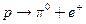Chapter 33, Problem 34PE

Chapter
Section
Textbook Problem

(a) Show that the conjectured decay of the proton,violates conservation of baryon number and conservation of lepton number.(b) What is the analogous decay process for the antiproton?

To determine

(a)

To show that the decay pπ0+e+ violates the conservation of baryon number and lepton

Number.

Explanation

Given info:

Given reaction is pπ0+e+.

Calculation:

We can check whether the decay pπ0+e+ violates the conservation of baryon number

and lepton number.

Decay:

pπ0+e+

B:  +1  0  0

To determine

(b)

the decay process of antiproton analogous to the reaction pπ0+e+.

Still sussing out bartleby?

Check out a sample textbook solution.

See a sample solution

The Solution to Your Study Problems

Bartleby provides explanations to thousands of textbook problems written by our experts, many with advanced degrees!

Get Started

Find more solutions based on key concepts